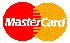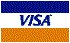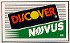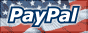Quintessential Oils

Weight & Measure Conversion Table

cc (cubic centimeters) = ml (millileters)
1/30 oz. = 1 ml = 20 drops
1/12 oz. = 2.5 ml = 50 drops
1/8 oz. = 3.75 ml = 75 drops
1/4 oz. = 7.5 ml = 150 drops
1/2 oz. = 15 ml = 300 drops = 1 tablespoon
1 oz. = 30 ml = 600 drops = 2 tablespoons
2 oz. = 60 ml = 1200 drops = 4 tablespoons
4 oz. = 120 ml = 2400 drops = 8 tablespoons = 1/2 cup
8 oz. = 240 ml = 4800 drops = 16 tablespoons = 1 cup
16 oz. = 480 ml = 9600 drops = 32 tablespoons = 2 cups = 1 pint
32 oz. = 960 ml = 19200 drops = 64 tablespoons = 4 cups = 2 pints = 1 quart
64 oz. = 1920 ml = 38400 drops = 128 tablespoons = 8 cups = 4 pints = 2 quarts
128 oz. = 3840 ml = 76800 drops = 256 tablespoons = 16 cups = 8 pints = 4 quarts = 1 gallon

*Drops are approximate depending on the differing specific gravity (weights) of oils. Some oils are thin and light (Lavender & Tea Tree, etc) while other oils are thick and heavy (Sandalwood & Patchouli, etc.).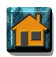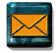To Place An Order: Phone: 510-215-2750 or Fax: 510-215-0278 or Email Form